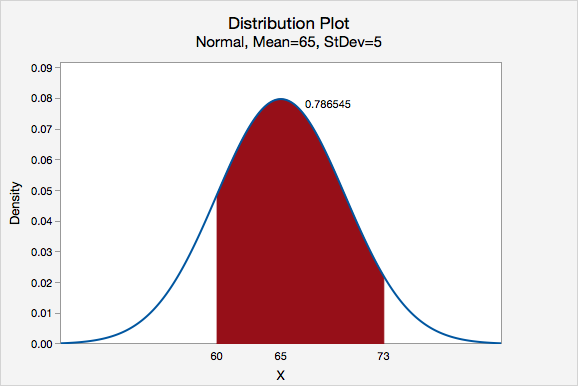# 7.2.3 - Proportion 'In between'

## MinitabExpress – Proportion Between Two z Values

Question: What proportion of the standard normal distribution is between a z score of 0 and a z score of 1.75?

Recall that the standard normal distribution (i.e., distribution) has a mean of 0 and standard deviation of 1. This is the default normal distribution in Minitab Express.

Steps
1. On a PC: from the menu select STATISTICS > Distribution Plot
On a Mac: from the menu select Statistics > Probability Distributions > Distribution Plot
2. Select Display Probability (Note: The default is the standard normal distribution)
3. Select A specified X value
4. Select Middle
5. For X value 1 enter 0 and for X value 2 enter 1.75

This should result in the following output:The proportion of the z distribution that is between 0 and 1.75 is 0.459941

Video Walkthrough

Select your operating system below to see a step-by-step guide for this example.

## MinitabExpress – Proportion Between Values on a Normal Distirbution

Question: Vehicle speeds at a highway location have a normal distribution with a mean of 65 mph and a standard deviation of 5 mph. What is the probability that a randomly selected vehicle will be going between 60 mph and 73 mph?

Let's construct a normal distribution with a mean of 65 and standard deviation of 5 to find the area between 60 and 73.

Steps
1. On a PC: from the menu select STATISTICS > Distribution Plot
On a Mac: from the menu select Statistics > Probability Distributions > Distribution Plot
2. Select Display Probability
3. For Distribution select Normal
4. For Mean enter 65
5. For Standard deviation enter 5
6. Select A specified X value
7. Select Middle
8. For X value 1 enter 60 and for X value 2 enter 73

This should result in the following output:On a normal distribution with a mean of 65 and standard deviation of 5, the proportion between 60 and 73 is 0.786545

In other words, 78.6545% of vehicles will be going between 60 mph and 73 mph.

Video Walkthrough

Select your operating system below to see a step-by-step guide for this example.